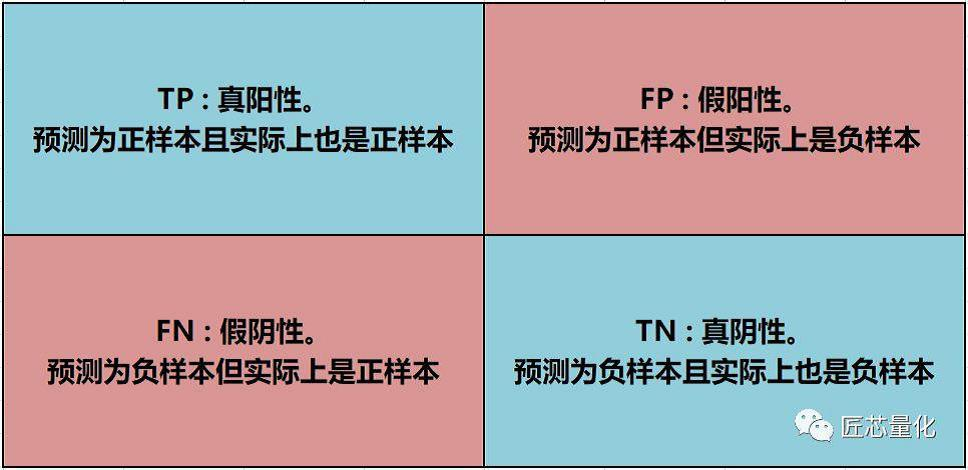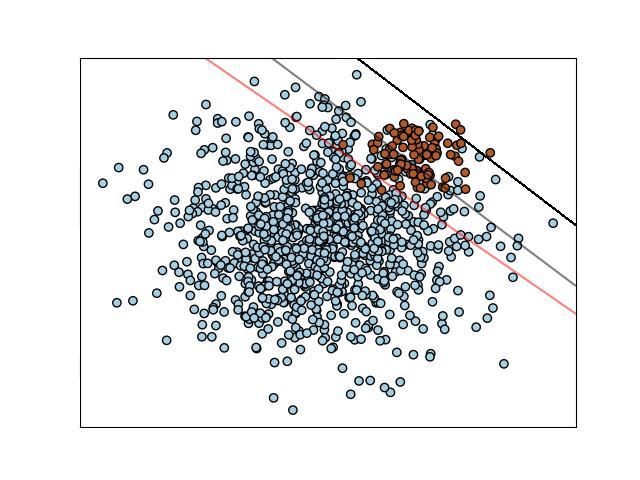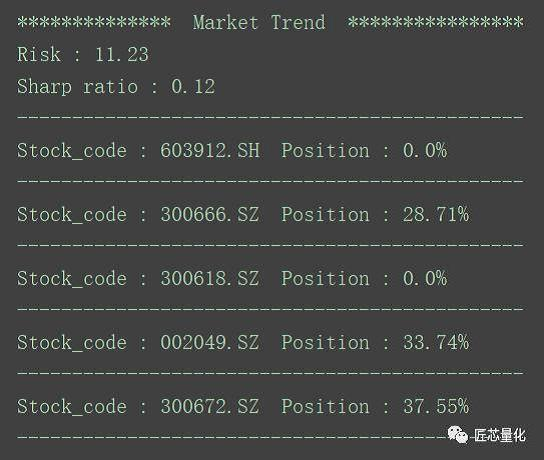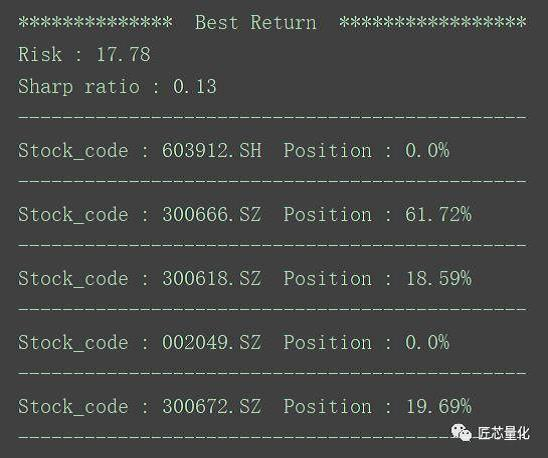## 机器量化分析（二）——模型评估与仓位管理

### >> 模型评估 <<

• 1. Acc（Precision） = Tp（预测为上涨且正确的次数）/ Tp + Fp(预测为上涨但实际不上涨的次数)。 该公式的分母，是所有预测为上涨（无论实际上涨与否）的次数。
• 2. Acc（Recall） = Tp（预测为上涨且正确的次数）/ Tp + Fn(预测不上涨但实际上涨的次数)。 该公式的分母，是所有实际上涨（无论预测上涨与否）的次数。F1 = ( 2 * Precision * Recall ) / ( Precision + Recall )

state_dt varchar2(45) 评估日期
stock_code varchar2(45) 股票代码
acc decimal(20, 4) 查准率
recall decimal(20, 4) 查全率
f1 decimal(20, 4) f1分值
acc_neg decimal(20, 4) 查准率（负样本）
bz varchar2(45) 用于标注模型类别，比如svm、决策树等
predict varchar2(45) 对评估日后一个交易日的预测值

state_dt varchar2(45) 回测日期
stock_code varchar2(45) 股票代码
resu_predict decimal(20, 2) 预测值
resu_real decimal(20, 2) 真实值

``````# 计算查全率
sql_resu_recall_son = "select count(*) from model_ev_mid a where a.resu_real is not null and a.resu_predict = 1 and a.resu_real = 1"
cursor.execute(sql_resu_recall_son)
recall_son = cursor.fetchall()
sql_resu_recall_mon = "select count(*) from model_ev_mid a where a.resu_real is not null and a.resu_real = 1"
cursor.execute(sql_resu_recall_mon)
recall_mon = cursor.fetchall()
recall = recall_son / recall_mon
# 计算查准率
sql_resu_acc_son = "select count(*) from model_ev_mid a where a.resu_real is not null and a.resu_predict = 1 and a.resu_real = 1"
cursor.execute(sql_resu_acc_son)
acc_son = cursor.fetchall()
sql_resu_acc_mon = "select count(*) from model_ev_mid a where a.resu_real is not null and a.resu_predict = 1"
cursor.execute(sql_resu_acc_mon)
acc_mon = cursor.fetchall()
if acc_mon == 0:
acc = recall = acc_neg = f1 = 0
else:
acc = acc_son / acc_mon
``````

• 1. 建回测时间序列。
• 2. 进行第一次时间序列的遍历，推进式建模，向中间表存入相关过程变量（包含每次的预测值）。
• 3. 进行第二次时间序列的遍历，向中间表中更新每次迭代的真实值。
• 4. 对中间表进行统计，计算Precision，Recall，F1分值等，并存入结果表。

（完整的模型评估代码详见 Model_Evaluate.py 文件）

### >> 仓位管理 <<

603912 佳力图 通用设备/5G/次新股
300666 江丰电子 半导体/芯片/次新股
300618 寒锐钴业 有色金属/锂电池
002049 紫光国芯 半导体/5G/两融股
300672 国科微 半导体/芯片/次新股

• 1. 计算投资组合在采样区间内的每日收益率，组成一个 m*n 的矩阵A（其中m为交易日天数，n为投资组合股票或基金数，m必须大于n）。
• 2. 计算该矩阵A的协方差矩阵
• 3. 求解该协方差矩阵的特征值和特征向量
• 4. （可选） 计算夏普率（完整的模型评估代码详见 Portfolio.py 文件）